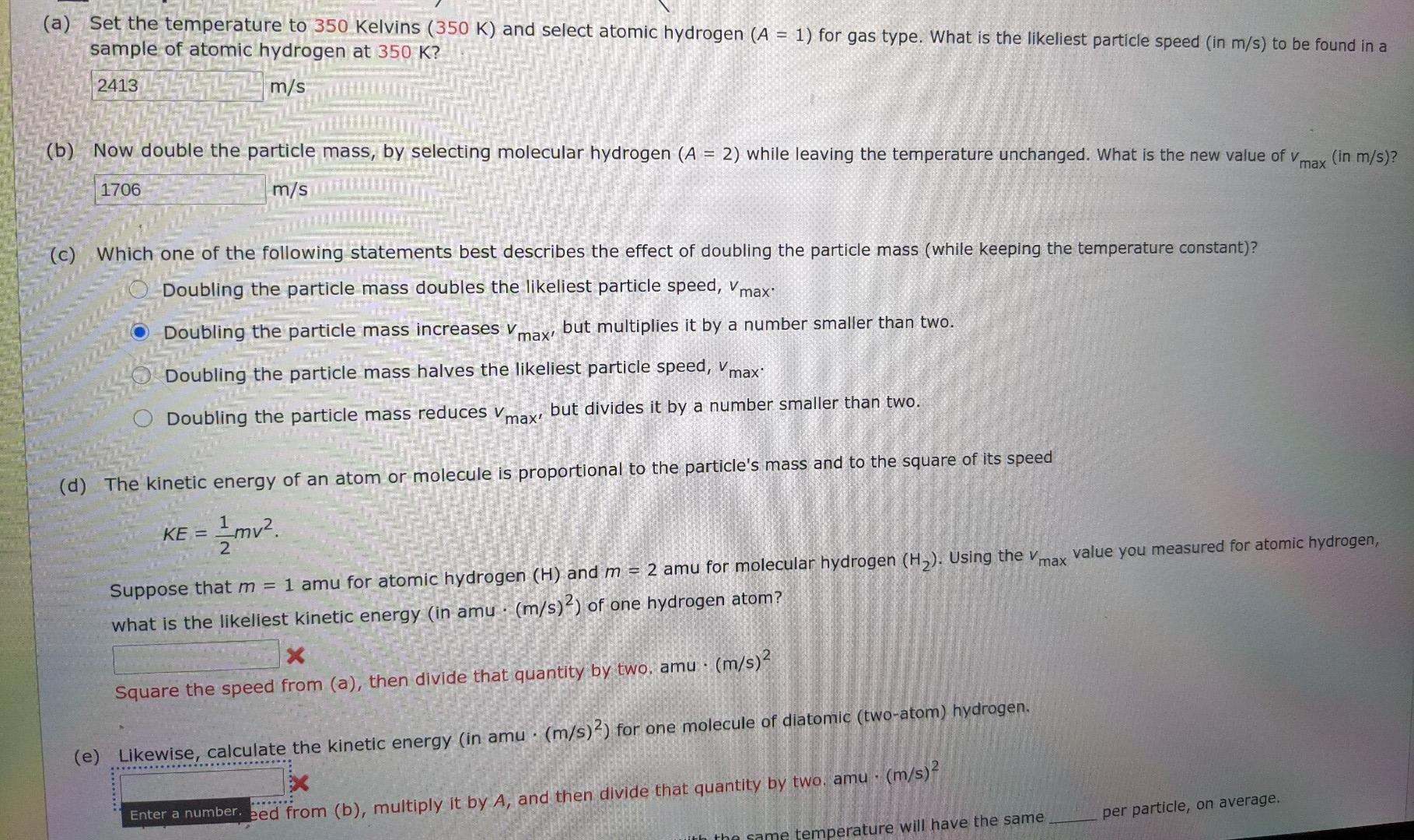# Question How do you solve d&e . I can't figure it out..when I breakdown the problem ..you would use 2413 for D and 1706 for e. using the kinetic energy formula to figure out the amu. (a) Set the temperature to 350 Kelvins (350 K) and select atomic hydrogen (A = 1) for gas type. What is the likeliest particle speed (in m/s) to be found in a sample of atomic hydrogen at 350 K? 2413 m/s (b) Now double the particle mass, by selecting molecular hydrogen (A = 2) while leaving the temperature unchanged. What is the new value of v (in m/s)? max 1706 m/s (c) Which one of the following statements best describes the effect of doubling the particle mass (while keeping the temperature constant)? Doubling the particle mass doubles the likeliest particle speed, Vmax. Doubling the particle mass increases v max' but multiplies it by a number smaller than two. Doubling the particle mass halves the likeliest particle speed, Vmax. Doubling the particle mass reduces Vmax, but divides it by a number smaller than two. (d) The kinetic energy of an atom or molecule is proportional to the particle's mass and to the square of its speed KE = 2 2 mu? Suppose that m = 1 amu for atomic hydrogen (H) and m = 2 amu for molecular hydrogen (H2). Using the v value you measured for atomic hydrogen, max what is the likeliest kinetic energy (in amu · (m/s)2) of one hydrogen atom? X Square the speed from (a), then divide that quantity by two, amu · (m/s)? (e) Likewise, calculate the kinetic energy (in amu · (m/s)?) for one molecule of diatomic (two-atom) hydrogen. Enter a number, eed from (b), multiply it by A, and then divide that quantity by two. amu (m/s)? per particle, on average. the same temperature will have the same